# python画散点图-python中画散点图

import numpy as np

import matplotlib.pyplot as plt

from mpl_toolkits.mplot3d import Axes3D

data = np.random.randint(0, 255, size=[40, 40, 40])

x, y, z = data, data, data

ax = plt.subplot(111, projection='3d') # 创建一个三维的绘图工程# 将数据点分成三部分画，在颜色上有区分度ax.scatter(x[:10], y[:10], z[:10], c='y') # 绘制数据点ax.scatter(x[10:20], y[10:20], z[10:20], c='r')

ax.scatter(x[30:40], y[30:40], z[30:40], c='g')

ax.set_zlabel('Z') # 坐标轴ax.set_ylabel('Y')

ax.set_xlabel('X')

plt.show()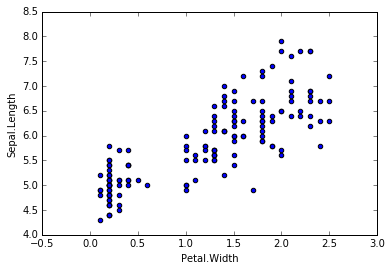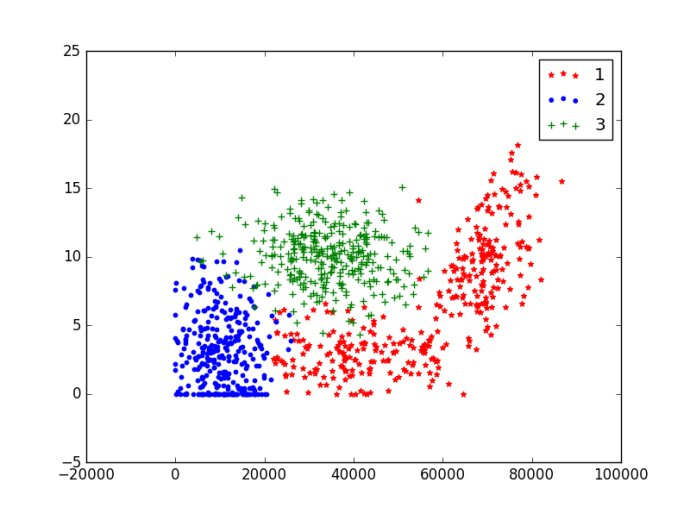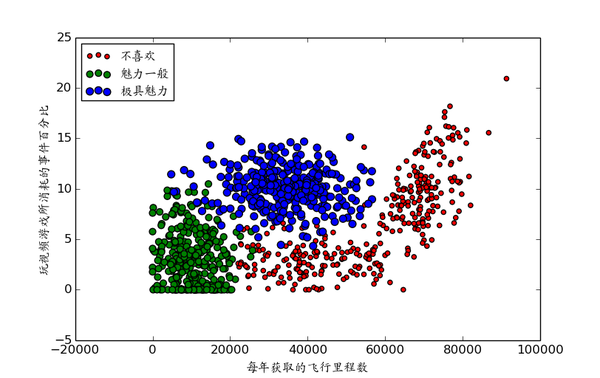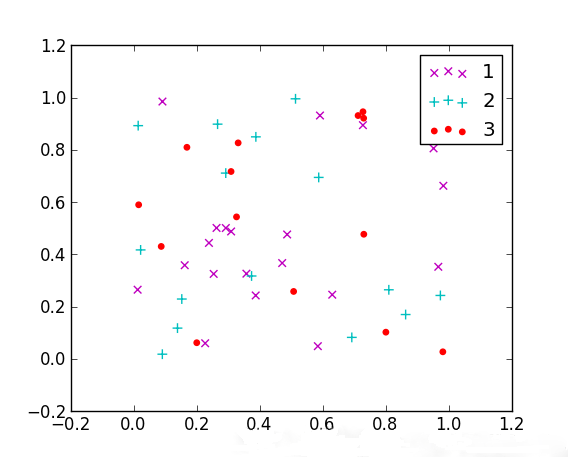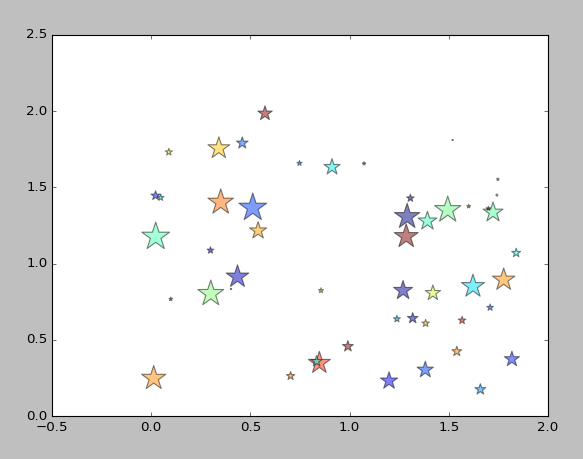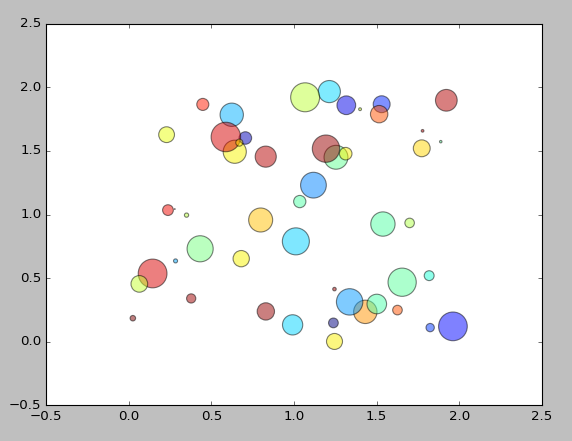Y形的散点图：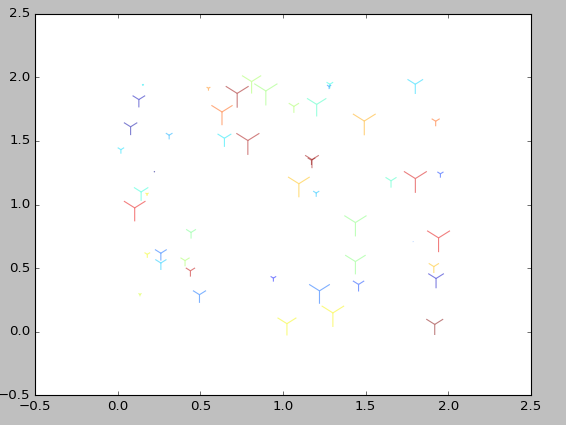from numpy import *;

N = 50 # 点的个数

x = np.random.rand(N) * 2 # 随机产生50个0~2之间的x坐标

y = np.random.rand(N) * 2 # 随机产生50个0~2之间的y坐标

colors = np.random.rand(N) # 随机产生50个0~1之间的颜色值

area = np.pi * (15 * np.random.rand(N))**2 # 点的半径范围:0~15

# 画散点图

plt.scatter(x, y, s=area, c=colors, alpha=0.5, marker=(9, 3, 30))

plt.show()

matplotlib.pyplot.scatter(x, y, s=20, c=None, marker='o',

cmap=None, norm=None, vmin=None, vmax=None, alpha=None,

linewidths=None, verts=None, edgecolors=None, hold=None,

data=None, **kwargs）

markerresult

”.”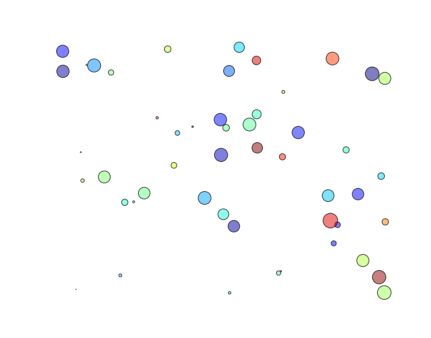”,”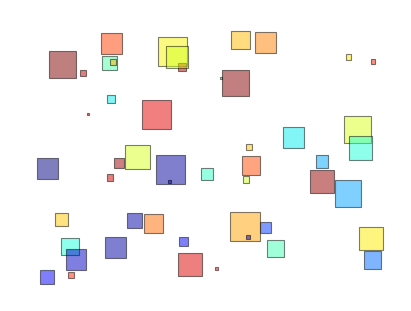"o”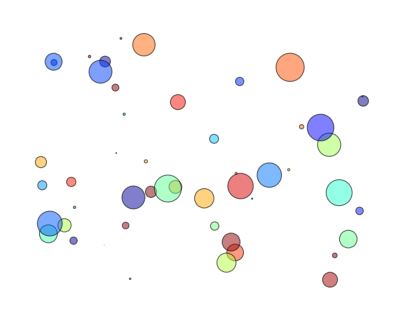"v”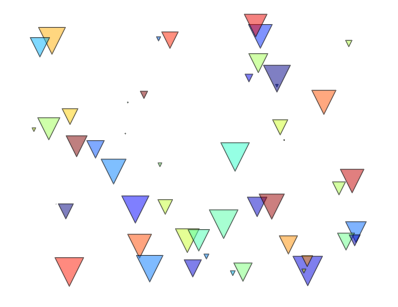"^”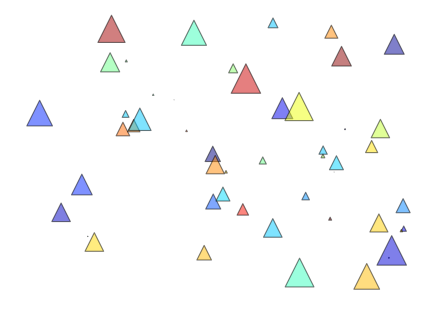"<”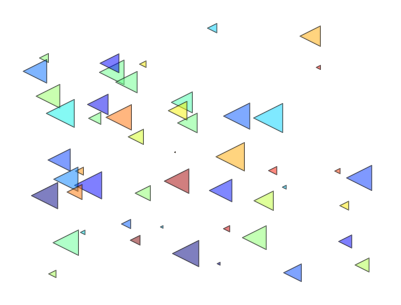">”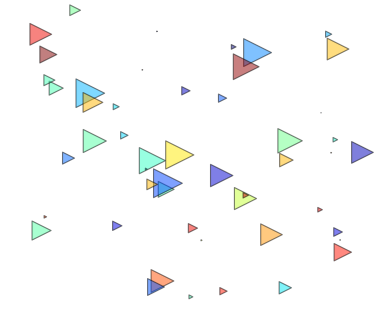"1”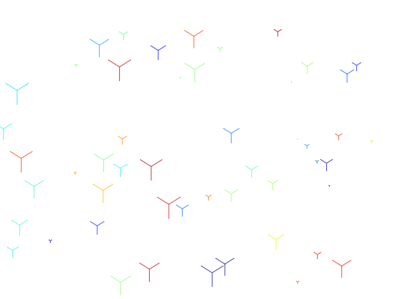"2”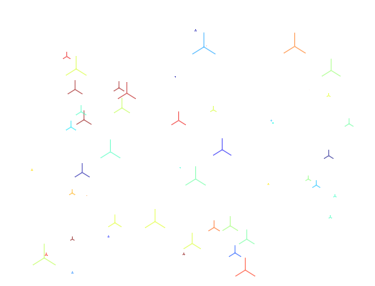"3”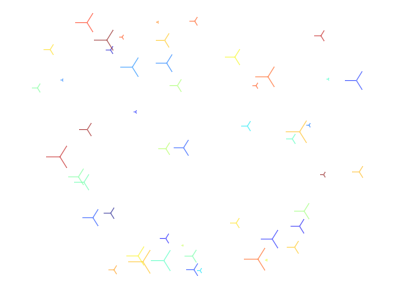"4”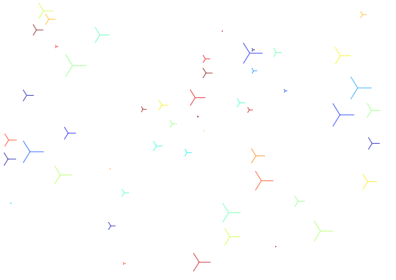"8”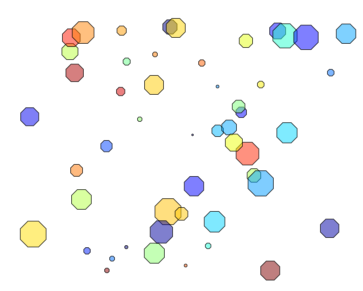"s”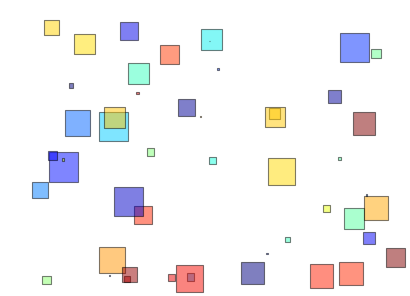"p”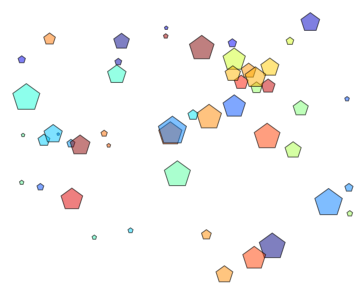"*”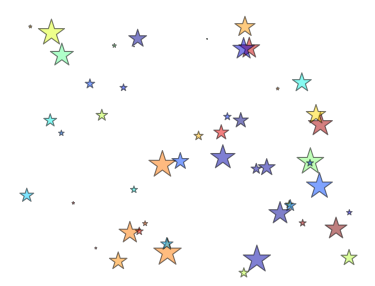"h”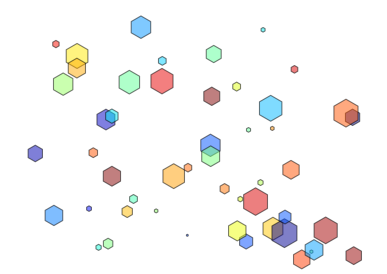"H”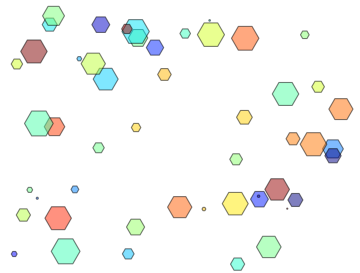"+”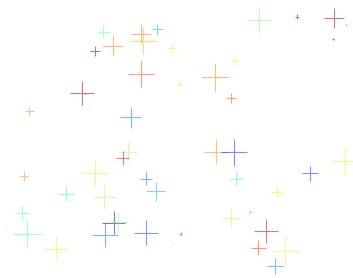"x”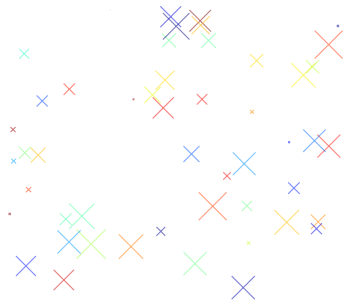"D”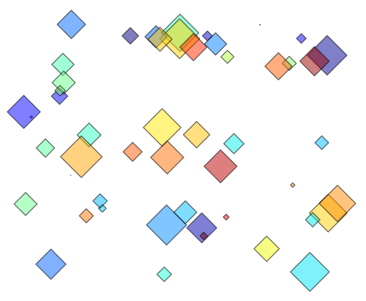"d”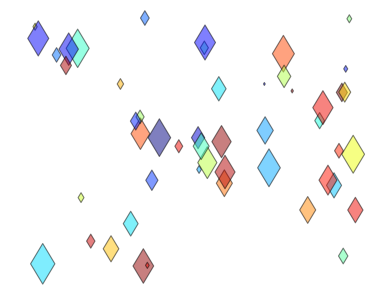"

"_”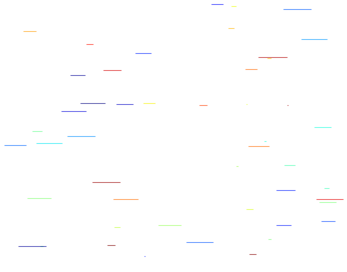"None”"$…$”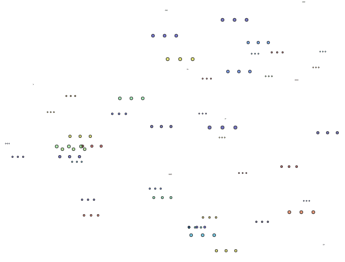(numsides, style, angle)

eg:(9,0, 30)

angle是旋转角度，

style只有0,1,2,3四个值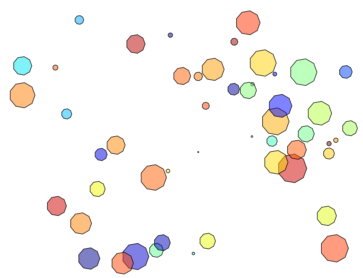(numsides, style, angle)

eg:(9,1, 30)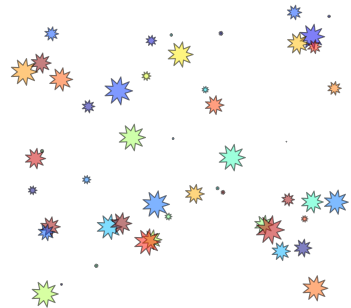(numsides, style, angle)

eg:(9,2, 30)(numsides, style, angle)

eg:(9,3, 30)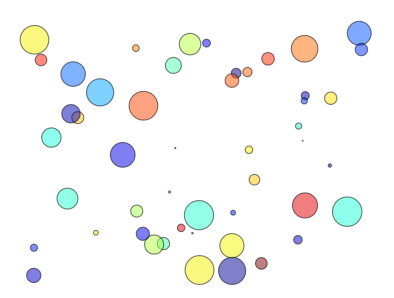……

09-081041
11-01241806-174092
01-285295
01-1818万+
08-016768
07-1310万+
11-304万+
02-17180
12-181421
10-281810
01-29641
07-305002
07-194797
01-06380
11-012944
11-01949
02-02708
04-13754
11-24131点击重新获取扫码支付余额充值